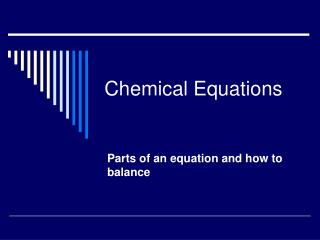DownloadDownload PresentationChemical Equations

# Chemical Equations

Download Presentation## Chemical Equations

- - - - - - - - - - - - - - - - - - - - - - - - - - - E N D - - - - - - - - - - - - - - - - - - - - - - - - - - -
##### Presentation Transcript

1. Chemical Equations Parts of an equation and how to balance

2. Chemical Equation: Set of directions for a chemical reaction. The directions tell us: • What materials to use • How much you need • Amount of product you will get

3. Chemical Equations * Give info about: • Reactants – substances being used in the reaction (starting materials on Left side of equation) • Products – new substances formed (ending materials on Right side of equation)

4. Numbers in Equations * • Coefficients – small whole numbers in front of compounds ex. 2 NaCl = 2 NaCl ’s • Subscripts – small numbers following elements ex. H2O = 2 H’s , 1 O

5. Indications of a Chemical Reaction * • Color • Gas / Bubbles • Precipitate • Temperature Change • Light Chemists Get Practice Trying Labs (Typically, more than one will be seen)

6. Diatomic Elements * • Two elements that exist bonded together when not bonded to another elements • Remember the seven + one rule that starts at element #7 H N, O, F Cl Br I At

7. Chemical Equation Symbols * SymbolMeaning (s) solid (l) liquid (g) gas (aq) aqueous dissolved in water

8. Chemical Equation Symbols * (Cont.) SymbolMeaning yields equilibrium heated temp at which reaction is run 3500

9. Balancing Equations • Check for diatomics • Balance metals • Balance nonmetals • Balance Oxygen • Balance Hydrogen • Recount all atoms • If every coefficient will reduce, write in simplest whole number ratio